# DC/DC converter datasheets - Calculate system losses

Other Parts Discussed in Post: LM2673

Welcome back to the DC/DC converter datasheets blog series. In this final installment, I will discuss DC/DC regulator component conduction losses.

Conduction losses are a result of device parasitic resistances impeding the DC current flow in a DC/DC converter. These losses are in direct relationship with the duty cycle. When the integrated high-side MOSFET turns on, the load current flows through it. The drain-to-source channel resistance (RDSON) causes power dissipation, which can be expressed as Equation 1: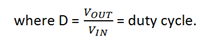For nonsynchronous devices like the LM2673, the diode is forward-biased when the integrated MOSFET turns off. During this time, the inductor current ramps down through the output capacitors, the load and the forward-biased diode. Since the load current is now conducting through the diode, there will be power dissipation in it, expressed as Equation 2: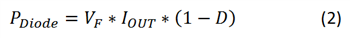Where, VF is the forward voltage drop of the chosen diode.

In addition to the conduction loss in the integrated MOSFET and the catch diode, there are conduction losses in the inductor as well because every inductor has a finite DC resistance (DCR), which is the resistance of the wire in the coil. Equation 3 expresses the dissipation in the inductor as: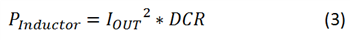The conduction losses depend on the load current. With heavier loads, the conduction loss in the MOSFET increases and is the dominating factor. Conduction losses plus switching, driver and internal low-dropout regulator (LDO) losses lead to a considerable generation of heat and increase the junction temperature of the integrated circuit (IC). Equation 4 expresses the increase in junction temperature as:where ICTj is the junction temperature of the IC, TA is the ambient temperature, θJA is the junction-to-air thermal resistance and ICPd is the total power dissipation just in the IC equal to .

The RDSON of the MOSFET typically has a temperature coefficient (RdsonTco). With an increase in the junction temperature of the IC, the RDSON would increase above the nominal based on the temperature coefficient. While this parameter may not be available in the data sheet, TI’s WEBENCH® Power Designer software has that information and uses it for calculating the design efficiency, thus making the results accurate. Equation 5 can adjust the RDSON based on the junction temperature:where RdsonNom is the nominal value of RDSON as listed in the data sheet.

The increase in RDSON depends on how well the device is heat-sinked and on the junction temperature of the device. Improper heat-sinking can lead to a drastic increase in RDSON and cause a larger dip in efficiency at full load. This is evident in situations when the die attach paddle (DAP) of the IC is not well soldered to the board.

Calculating losses is an iterative process. You will need to evaluate the junction temperature and the resulting RDSON on every iteration of calculating the IC power loss to get accurate results in efficiency. WEBENCH Power Designer takes care of this; it displays the calculated results of passive component losses as well. Knowing these losses is important because it will help you choose the right components, along with a DC/DC regulator that will help maintain good efficiency. The total conduction losses can now be expressed as Equation 6: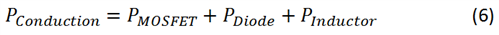Looking over all of the losses, Equation 7 adds them up to calculate the total loss: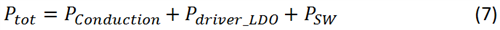Equation 8 expresses the resulting efficiency of the DC/DC regulator design as:Figure 1 shows the overall efficiency against the load-current curves of the LM2673 taken at different input voltages. You can see that at light loads the efficiency deteriorates; from parts 1 and 2 of this series, you know to attribute this to the switching losses and the losses in the driver and LDO, respectively. Also notice that at the maximum load current, the efficiency at a higher input voltage (VIN) is lower because of the higher switching losses that occur at such voltages. The low VIN efficiency at loads above 1A is comparatively higher because of the reduced switching losses.

This ends my three-part blog series on reading and understanding efficiency in data sheets. Now you should be able to understand the different component losses that take place in a DC/DC regulator design. Based on your application needs, you can now make informed decisions when selecting DC/DC regulators, their switching frequency, amount of required board space for heat-sinking, and also when selecting passive components like the diode and the inductor. Select a SIMPLE SWITCHER DC/DC regulator and start a design now in WEBENCH Power Designer.